# Work, Energy and Power

 This page gives a background to some of the clips shown in the multimedia tutorial.We all know about physical work, so we started the tutorial with this example, which also gives an idea of the size of the quantities involved. We begin with the calculations behind the histograms we showed. These are 20 kg bags so the weight of each is about 200 newtons down, which is the grey arrow. Normally we don't say 'down' in this context, because weight is always in a direction close to down. I did so here to remind you that weight is a vector. So let's write, for one bag, W = − mgj = − (200 N)j. Check that notation: weight, W, is a vector, whereas work, W, is a scalar. (Occasionally we shall also need W for the magnitude of W, but you will know from context which is which.) The motion of the bags is slow, their accelerations are small compared to g, so the force required to accelerate them is small compared to their weight. So when I lift them, I'm applying a force F ≅ − W = (200 N)j, which is the black arrow. If you remember the scalar product, you'll know that i.j = 0 but j.j = 1. So, if I apply this constant force over a displacement Δs = Δxi + Δyj, the work (W) I do is W  =  F.Δs  =  (mgj).(Δxi + Δyj)  =  mg Δy (which, as we show in the multimedia tutorial, is the increase in potential energy of a mass m in a gravitational field of magnitude g when raised a height Δy). So, for the first bag, the force is 200 N, I lift it about 0.7 m (the red arrow), so I do 140 J of work. The joule (symbol J) is the SI unit of work: one newton times one metre. The joule is not very big on a human scale: lift a small apple (weight about 1 N) through a height of 1 m and you've done 1 joule of work on the peach – but rather more than that in moving your arm! Similarly, although I only do 140 J on the bag, I do more work on moving my arms and torso. It's possible for a fit person to do a megajoule of work in an hour.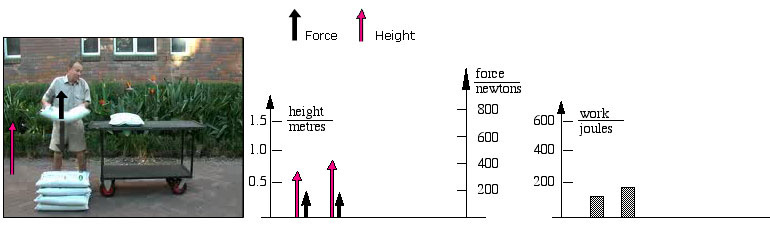The pile is now shorter so I must lift the second bag through a larger increase in height: which reminds me that work is proportional to displacement!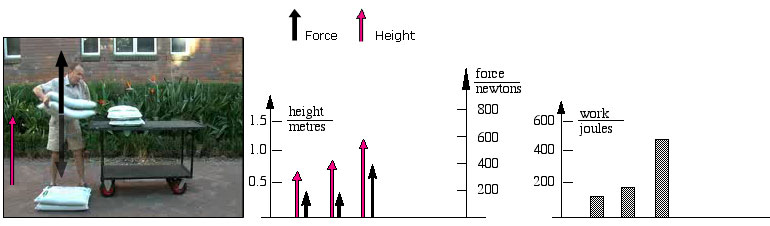Work is also proportional to the force, so lifting two bags requires twice the force and I do twice as much work as on the first bag.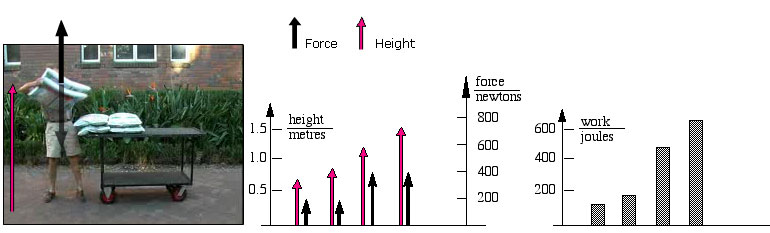Hmm, I've not planned this well and must lift the last two bags further: 400 N times 1.5 m is 600 J.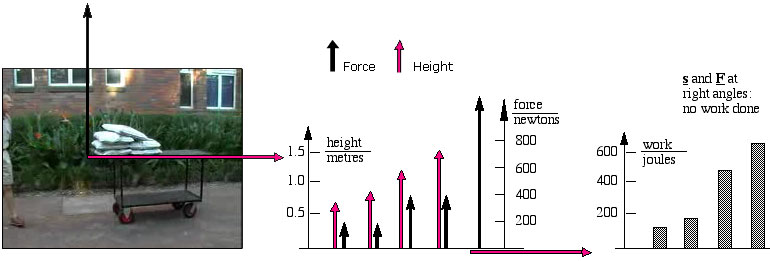Look at the big displacement of the trolley and how easy it is. The trolley is supporting six bags, so the force it applies upwards has magnitude 1.2 kN (black arrow), and it moves several metres (red arrow). But the force is in the j (or y) direction and the displacement in the i (or x) direction: they are at right angles. Remember i.j  =  0.   Or    F.Δcos θ  =  0.So no work is done.
 Lifting 20 kg bags (weight = 200 N) is not so hard. Lifting my own 70 kg mass (weight W = 700 N)requires more force. But not if we use pulleys (which are discussed in more detail in the physics of blocks and pulleys). Here, a single rope goes from the support, down to my harness, round the pulley, back to the support, round another pulley and back to my hands. The pulleys turn easily, so the tension T in each section of the rope is the same. There are three sections pulling me upwards. From Newton's second law, the total force acting on me equals my mass times my acceleration. Compared with g, my acceleration here is negligible. So 3T + W  =  ma  ≅  0 So, if we neglect my (modest) acceleration, the force of the three sections pulling upwards on me equals my weight: the magnitude of the tension is T = |W|/3 = (700 N)/3. So the force I need supply with my arms is reduced. However, to lift my body through say 2  m in altitude, I must still do (700 N)(2 m) = 1.4  kJ of work. How is this possible? Well, each of the three sections of rope shortens by 2  m. So my hands pull the rope 6  m. I do work (6  m)(700 N)/3 = 1.4  kJ of work (plus a bit extra to overcome friction in the pulleys). Like levers, blocks and pulleys don't save you work, but they can reduce (or increase) the force, which can make a task more convenient and comfortable.### Kinetic energy and the work energy theorem

The multimedia tutorial presents this theorem, but perhaps you'd like to see it again here. Let's apply a constant force F to a mass m as it moves, in one dimension, a distance x. (It might, for instance, be the magnetic force that we used in our section on Newton's laws.) The force is constant over x, x increases linearly over x, so the work done ∫ F.dx increases linearly over x.
 Once we relate this to time and velocity, we shall have to do the integration. (Remember that there is help with calculus.) So let's consider the case – still one dimensional – in which the force is applied over a short distance dx, and that the mass m increases in velocity from v to v+dv.
 The total work done on the mass is dW  =  Fdx where F is the total force acting on the mass. Subsituting for Newton's second law, F = ma = m(dv/dt), gives: dW  =  m(dv/dt)dx  =  m*dx*dv/dt where we have written the mutliplication and division explicitly. dx, dv and dt are all small quantities, but there is no reason why we cannot change the order of multiplication. So let's write: dW  =  m*dv*dx/dt  =  m*v*dv The advantage of this rearrangment is that we can now do the integral easily: W  =  ∫ dW  =  ∫ mv.dv Suppose we start from v = 0, then the total work done to accelerate mass m from rest to a speed v is: W  =  ∫ mv.dv  =  ½mv2 This quantity is so useful that we give it a name, the kinetic energy and write K  =  ½mv2
 So you don't like calculus? Let's use the equations from one-dimensional kinematics (for which there is a multimedia tutorial). Let's suppose that a body starts from rest and that we apply a constant (total) force F for a certain time T in one example, and for twice that time (2T) in a later example (the black graphs at right). The final velocity will be v = aT = (F/m)T in the first example, and twice that value in the second example (the red graphs at right). The distance travelled while the force is acting, i.e. the distance travelled during the acceleration, is now four times as great, as shown in the purple graphs at right. So the constant force has been applied over four times the distance, and has done four times the work. So, even though the velocity has only doubled, we have done four times as much work (blue graph at right). That is an important consequence: at twice the speed, a mass has four times the kinetic energy. This has important implications for road safety, as we see next.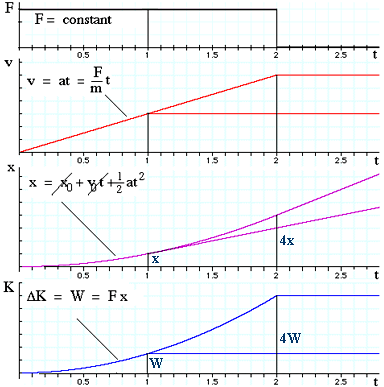### Stopping distances and the work energy theorem

If I travel twice as fast on my bicycle, how much further does it take to stop? (I include only the distance after I apply the brakes, not the time it takes me to react to danger and to apply the brakes.
At twice the speed, my kinetic energy K = ½mv2 is four times as great. So, to do four times as much (negative) work, the braking force (assumed constant) must be applied over four times the distance. Please remember this on the road.

### Potential energy

Suppose I slowly lift a mass in a gravitational field. In this clip from the multimedia tutorial, the rope, with a little assistance from me, is slowly lifting a container of water. The tension force F is doing work W on the container, but it is not increasing its kinetic energy. The reason, of course, is that the weight mg of the container is pulling the other way: it is doing negative work on the container. However, the work W is not lost: we can recover it: we can slowly lower the container, and thus lift the the brick on the other end of the rope.

So where is the work done by F going when we lift the container? It is, in a sense, stored in the gravitational interaction between the container and the earth. This 'stored work' has the potential to do work for us. This is an example of potential energy – in this case gravitational potential energy. So, how much potential energy do we store in this case?

We use a force F to move an object of mass m a displacement ds in a gravitational field, so we do work

dW  =  F.ds ,

(where you might wish to revise vectors). Suppose that we are moving it in such a way that we do not change its velocity (and so don't change its kinetic energy). Then the total force on it is zero, so F + mg = 0, so

dW  =  −mg.ds .

However, g is in the negative vertical direction, say the minus y direction, so

dW  = mgdy.

For displacements on the planetary scale, we'd have to consider the variation of the gravitational field with height, which we do in the section on gravity. For more modest displacements, g is uniform and integration gives us

∫ dUgrav  =  ∫ dW  =  ∫ mg dy  =  mgΔy  =  mgΔh

where h is commonly used for the vertical coordinate. U is defined by an integral, and integrals require a constant of integration. For potential energy, this constant is the reference for the zero of potential energy. If we define Ugrav to be zero at h = 0, then we can write

Ugrav = mgh.

As we shall see, not all forces allow one to define a potential energy. However, another example is the#### Potential energy of a spring.

If we slowly compress or extend a spring from its resting position, again we do work without creating kinetic energy. But again it is 'stored' – we can get it back. From Hooke's law, the force exerted by a spring is Fspring = − kx, where x is the displacement from its unstretched length, and k is the spring constant for that particular spring. Because we are not accelerating anything, we have to apply a force F = − Fspring, so

∫ dUspring  = ∫ dW  =  ∫ F dx  =  −∫ Fspringdx  =  ∫ kx dx =  Δ(½kx2)

Again, we have a constant of integration and a zero of potential energy to define. Usually, we set U = 0 at x = 0, so

Uspring  = ½kx2.

Note that, with this reference value, Uspring is always positive: with respect to the unstressed state, both stretching (x > 0) and compressing (x < 0) require work, so the potential energy is positive in each case.

In the film clip, I do work to store potential energy in the spring, the spring then does work on the mass, giving it kinetic energy. Biochemical energy in my arm was converted into potential energy in the spring and then to kinetic energy.

### Conservative and non-conservative forces

Let's look at the work that I do in moving a mass in a gravitational field. We'll pretend that I do this with accelerations so slow that the mass is always in mechanical equilibrium, i.e. that the force exerted by my hand plus the weight of the mass add to zero, so

Fhand  =  −mg

The work I do against gravity is ∫ Fhand.ds, which is shown as the brown coloured histogram.

As I lift the mass, Fhand is upwards (positive) and s is also positive, so the work done by me is positive:

∫ Fhand.ds > 0.

As I lower the mass, Fhand is still upwards (positive) but now s is negative, so the work done by me is negative:

∫ Fhand.ds < 0.

Consequently, round a complete cycle that returns the mass to its starting point, ∫ Fhand.ds =0. Similarly, the work done by gravity around the cycle is zero (because Fgrav = −Fhand). This makes gravity a conservative force:

Definition: A conservative force is one that does zero work around a closed loop in space. It follows that, for a conservative force F, we may define a potential energy as a function of position r:

U = U(r) ≡ ∫ F.dr .

If the work done around a closed loop is not zero, then we cannot define such a function: its value would have to change with time if we went around such a loop. Forces with this property are called, obviously, nonconservative forces.

So, what sort of force is that exerted by an ideal spring?

 Again, let's imagine that I do this so slowly that the spring is in mechanical equilibrium: Fhand = −Fspring. I move my hand to the right, stretching the spring. Fhand is positive and ds is positive. I do positive work (shown in the histogram) and the spring does negative work. Then I move my hand to the left, but still pulling to maipntain the stretch in the spring. As the spring shortens, Fhand is still positive but now ds is negative. I do negative work (shown in the histogram) and the spring does positive work. For the spring, ∫ F.dr around a closed path is zero. The force exerted by an ideal spring is a conservative force. What sort of a force is friction?

Again, let's imagine that I do this so slowly that the mass is in mechanical equilibrium: Fhand = −Ffriction. Moving to the right, I apply a force to the right and the object moves to the right: Fhand and ds are both positive: I do positive work (shown in the histogram) and friction does negative work. Moving to the left, I apply a force to the left and the object moves to the left: Fhand and ds are both positive: I do positive work (shown in the histogram) and friction does negative work. So, around a closed loop, the work done against friction is greater than zero, so friction is a nonconservative force.

### Conservation of mechanical energy

We saw above in the work energy theorem that the total work ΔW done on an object equals the increase ΔK in its kinetic energy. But consider the case where all of the forces that do the work ΔW are conservative forces: here, the work done by those forces is minus one times the work done against them, in other words it is −ΔU. So, if the only forces that act are conservative forces, then ΔU + ΔK = 0.

Define the mechanical energy:    E ≡ U + K.

So , if the only forces that act are conservative forces, mechanical energy is conserved. We shall make this stronger below but, before we do let's look at an example in which mechanical energy is (nearly) conserved.

#### Kinetic and potential energy in the pendulum

This video clip shows an example of the exchange of kinetic and potential energy in a pendulum. A warning, however: for the sake of keeping the download time small, this film clips is a single cycle repeated. In the original film, the pendulum gradually loses energy: in each cycle, a small fraction of the energy is lost, partly in pushing the air out of the way.

The kinetic energy K is shown in red: as a function of x on the graph, and as a histogram that varies with time. Note that the K goes to zero at the extremes of the motion. The potential energy U is shown in purple. It has maxima at the extremes of the motion, when the mass is highest. Because the zero of potential energy is arbitrary, so is the zero of the total mechanical energy E = U + K. Here, E (shown in white) is constant.

The Waves and Sound section of Physclips has a chapter on the mechanics of Oscillations and a section on Mechanical energy in Simple Harmonic Motion.

### Conservation of mechanical energy: statement

We have seen that, if the only forces present are conservative, then mechanical energy is conserved. However, we can go further.  Provided that nonconservative forces do no work, then the increase ΔK in the kinetic energy of a body is still the work done by the conservative f orces, which is −ΔU. So we can conclude that

If nonconservative forces do no work then mechanical energy (E ≡ U + K) is conserved.
This statement can be written in several ways, of which here are two:
If nonconservative forces do no work,      ΔU + ΔK  =  0       or        U i+ Ki  =  Uf + Kf ,
where i and f mean initial and final. I strongly advise that you always write the qualifying clause because, in general, mechanical energy is not conserved. (And never, ever, write "kinetic energy equals potential energy". That is not true, and you shouldn't tell lies.)
 On a rolling wheel, friction does no work. Here, I'm travelling slowly so let's neglect air resistance and rolling resistance. There is a substantial friction force: it is friction between tires and paving that accelerates me in a circle. In this case, the frictional force force is at right angles to the displacement, so friction does no work. So, while I'm not pedalling, (approximately) no work is done and my mechanical energy is (approximately) constant.

### Work and power

 Power is defined as the rate of doing work or the rate of transforming or transferring energy: P = dW/dt. In this example, my kinetic energy is approximately constant. However, my potential energy is increasing. Because I'm climbing, I'm not going very fast, so the rate at which I'm doing work against nonconservative forces such as air resistance is small. The equations below allow us to calculate the rate at which I'm doing work against gravity (which is an underestimate of the rate at which I'm doing work). My altitude is increasing at 1 m.s−1, and my weight is 700 N, so P  =  dW/dt  ≅  dU/dt  =  mg(dh/dt)  =  700 W.

### The sliding problem

Here is the problem from the tutorial: doing work against a nonconservative force. Here I apply a force F via the tension in a string. The work dW that I do is

dW  =  F.ds  =  F ds cos θ
Now v = ds/dt, so the power I am applying, i.e. the rate at which I am doing work is:
P  =  dW/dt  =  F v cos θ
I'll leave it to the reader to draw a free body diagram. Then use Newton's second law, then relate P to m, g, v and μk.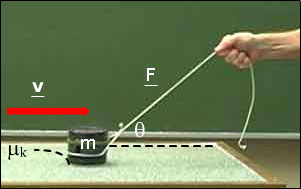### The loop-the-loop problem

This is a classic problem. A small toy car runs on wheels that turn are assumed to turn freely and whose mass is negligible, so we can treat it as a particle. From how high must I release it so that it will loop the loop, remaining in contact with the track all the way around?

If the car retains contact with the track then, at the top of the loop, which is circular, the centripetal acceleration will be downwards and its magnitude will be v2/r.

The forces providing this acceleration are its weight mg (acting down) and the normal force N from the track, also acting down at this point.

So, if N > 0, we require v2/r > g, or, for the critical condition at which it just loses contact, we require

vcrit2/r  =  g  or   vcrit2  =  rg

We can do this problem using the conservation of mechanical energy.
Uinitial + Kinitial  =  Ufinal + Kfinal
Choosing the bottom of the track as the zero for U, we could write,
mghinitial + 0  =  mg.(2r) + ½mvfinal
and, if vfinal = vcrit  =  √(rg)
mghinitial  =  2mgr + ½mgr
So the cricital height is 5r/2 above the bottom of the track.

### The hydroelectric dam problem

 The water level in a hydroelectric dam is 100 m above the height at which water comes out of the pipes. Assuming that the turbines and generators are 100% efficient, and neglecting viscosity and turbulence, calculate the flow of water required to produce 10 MW of power. The output pipes have a cross section of 5 m2. This problem has the work-energy theorem, uses power, and requires a bit of thought. Let's do it. Let's consider what is happening in steady state for this system. Over a time dt, some water of mass dm exits the lower pipe at speed v. This water is delivered to the top of the dam at negligible speed. So the nett effect is to take dm of stationary water at height h and deliver it at the bottom of the dam at height zero and speed v. Looks straightforward. Let's go.
Let the flow be dm/dt. The work done by the water, dW, is minus the energy increase of the water, so
dW  =  − dE  =  − dK − dU
=  − (½dm.v2 − 0) − (0 − dm.gh)  =  dm(gh − ½v2)
The power delivered is just P = dW/dt. so
P  =  (gh − ½v2)dm/dt

Of course the flow dm/dt depends on v. Let's see how: In time dt, the water flows a distance vdt along the pipe. The cross section of the pipe is A, so the volume of water that has passed a given point is dV = A(vdt). Using the definition of density, ρ = dm/dV, we have

dm/dt  =  ρdV/dt  =  ρA.(vdt)/dt   =  ρAv. Substituting in the equation above gives us

P  =   ρAv(gh − ½v2)   or

½v3 − ghv + P/ρA  =  0.

However you look at it, it's a cubic equation, which sounds like a messy solution. However, let's think of what the terms mean. The first one came from the kinetic energy term. The second is the work done by gravity. The third is the work done on the turbines. Now, if I had designed this dam, I'd have wanted to convert as much gravitational potential energy as possible into work done on the turbines, so I'd make the pipes wide enough so that the kinetic energy lost by the water outflow would be negligible. Let's see if my guess is correct.

If the first term is negligible, then we simply have hgv = P/ρA. So v = P/ρghA = 2 m.s−1. So the first term would be 4 m3.s−3, the second would be − 2000 m3.s−3 and the third would be 2000 m3.s−3. So yes, the guess was correct and, to the precision required of this problem, the answer is v = 2 m.s−1.

### Bernoulli's equation

Bernoulli's equation is an example of the work-energy theorem. In the animation, a fluid flows at a steady rate into a pipe with cross section A1 and height h1, where it has velocity v1 and pressure P1. The fluid leaves the pipe with cross section A2 and height h2, where it has velocity v2 and pressure P2. The fluid has constant density ρ and we assume that its viscosity is negligible, and that there is no turbulence, so that nonconservative forces do no work. What is the relation among the velocity, height and pressure? What is the relation among the velocity, height and pressure?

Before doing this quantitatively, we can ask how pressure and velocity are related. At the same height, and if we have no turbulence or viscosity, then the only thing that accelerates the fluid is the difference in pressure. The fluid will accelerate from high to low pressure so, where P is high, v should be low and vice versa. Let's see:

In a short time dt, a mass dm enters the pipe at left and, because the flow is steady, an equal mass dm flows out at right. Because the flow is steady, the total energy of the water in the pipe is unchanged. So the total work done on dm, by the work-energy theorem, is

dWtotal  =  ½ dm.v22 − ½ dm.v12.
The work is done by two forces: gravity, which does work − dUgrav, and the pressure. dUgrav is dm.gΔh, so
dWpressure − dUgrav  =  ½ dm.v22 − ½ dm.v12 , so

dWpressure  =  ½ dm.v22 + dm.gh2 − ½ dm.v12  − dm.gh2 .

So, how much work is done by the difference in pressure acting across the pipe? By definition, the pressure is the force per unit area, so the force exerted by P on cross sectional area A is PA. If this force is applied over a distance ds at right angles to A, it does work PAds. But the volume moved is dV = Ads, so the work done by pressure is PdV. The work done by P1 is positive and that done by P2 is negative, so
P1dV − P2dV  =  ½ dm.v22 + dm.gh2 − ½ dm.v12  − dm.gh2 .
Now we use the definition of density: ρ = dm/dV. So, if we divide both sides of the equation by dV and rearrange the terms,
P1 + ½ρv12 + ρgh1  =  P2 + ½ρv22 + ρgh2.
Of course, we could apply this analysis to any two points in the pipe so, provided that the flow is steady, incompressible, non-viscous and non turbulent, we have Bernoulli's equation
P + ½ρv2 + ρgh  =  constant.

Remembering that ρ = dm/dV, we can see the significance of each of these terms: P is the work done by pressure, per unit volume, ½ρv2 is the kinetic energy per unit volume, and ρgh is the gravitational potential energy per unit volume. Bernoulli's equation is just the work energy theorem, written per unit volume. In the absence of flow, this just gives the variation of pressure with depth:

ΔP  =  − ρgΔh ,    if there is no flow.
If the height is constant, we have
ΔP  =  − Δ(½ρv2) ,    if there is no change in height.
This last observation tells us that (at equal height), pressure will be high when velocity is low and vice versa. This makes sense: if the velocity has increased at constant h, then pressure must have acted to accelerate the fluid. The fluid of course flows from high pressure to low, so it must be slower in high pressure and faster in low pressure. (With the reminder that we are neglecting viscosity and turbulence: there are no non-conservative forces acting.)

 This is a nice demonstration: the hose delivers a high speed jet of air. What is holding the ball up in the air? The drag of the air jet as it passes the ball makes it rotate, so we can deduce from the direction of rotation that most of the jet passes above the ball. The ball has weight, and the only forces acting on it are those due to the pressure of the air around it. So we can conclude that the pressure above the ball is substantially less than that below the ball. This is not, however, a simple demonstration of the effect described by Bernoulli's equation. It is certainly true that the fast moving air coming out of the hose has a pressure somewhat less than the pressure in the stationary air. Because the jet of air coming out of the hose is mainly deflected above the ball, this makes the pressure above the ball less than atmospheric. However, in this case the jet itself is deflected by the presence of the ball, so there is also a contribution from the change in momentum of the jet. (Further, the drag that causes the rotation tells us that there is a nonconservative force present and so Bernoulli's equation would not apply accurately here.)

### Centre of mass work

When we write W = ∫F.ds, for an extended object, what is F and what is ds?

F is the total external force acting on the object which, because of Newton's third law, equals the total force on the object. ds in this case is the displacement of the centre of mass, dsCoM. In this simple demonstration, the force that accelerates me is the force that the wall exerts on my hand. The wall, however, doesn't move. What does move during my acceleration is my centre of mass, so the kinetic energy associated with the motion of my centre of mass is increased by ∫Fexternal.dsCoM.

We'll leave the derivation of this to the section on centre of mass.

### Work done by friction

Friction is complicated, and calulations of the work done by friction involve some approximations and subtleties. Suppose we consider a block sliding on a surface at constant velocity, and that we treat the block as a point mass. If we look at the block alone, two forces act on it: the external applied force, which does work W, and friction from the surface. Because the object is not accelerating, these forces are equal and opposite, so the total work done on the block is zero and friction does work W on the block (same magnitude force, opposite direction over same displacement).

In the macroscopic picture, no work is done on the block, so from the work-energy theorem, its kinetic energy is unchanged. The –W work done by friction is converted to heat (and a tiny amount of sound and some plastic deformation of the surface). Here is one complication: in the frame of reference of the surface, the block is moving, so –W is done by the frictional force that the surface exerts on the block (which moves) and zero work is done by the frictional force that the block exerts on the lower surface (which doesnt move). Alternatively, in the frame of reference of the block, the surface is moving, so –W is done by the frictional force that the block exerts on the surface (which moves) and zero work is done by the frictional force that the surface exerts on the block (which doesn’t move). And in a symmetric reference frame moving at v/2 over the surface, –W/2 would be calculated for each.

In practice, much of –W becomes heat, parts of which are conducted into both, though not usually symmetrically. The microscopic picture it is considerably more complicated, because friction often involves transient spot adhesions of tiny points of contact and microscopic deformations of the two sides, with inhomogeneous creep motions and accelerations.

### Why muscles get tired without doing work

A student's question: If I hold up an object without moving it, I'm not doing work (by the physics definition), but I still get tired. Why is it so? The short answer is that muscles require biochemical energy to exert a force, even if there is no motion. So we use biochemical energy, which is tiring, even if the energy used does zero work. There's a separate page about this.This work is licensed under a Creative Commons License.